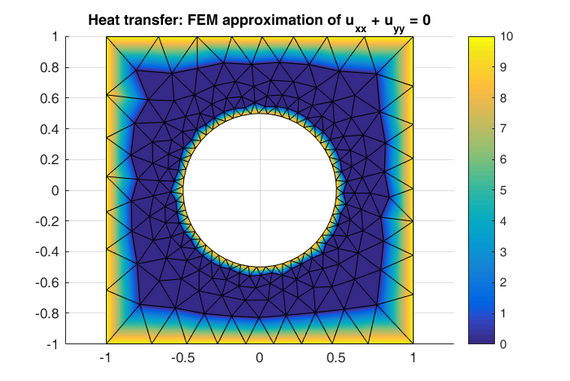## Numerical Methods

This Master module of the Mechanical Engineering/Mechatronics programme enables students to build mathematical models for common classes of engineering problems and solve the problems by implementing appropriately chosen numerical methods, with a focus on ordinary and partial differential equations. Students learn to transfer state-of-the-art numerical methods to practical problem-solving engineering solutions and present and discuss their solutions in group reviews.

### Content

Topics include

• Basic concepts of mathematical modeling and numerical analysis
• Numerical differentiation and integration
• Numerical solutions of ordinary and partial differential equations
• Finite difference method (FDM), finite element method (FEM)
• Unconstrained and constrained optimizationThe course description can also be found in the Campusboard-platform of the university.

### Learning goals

Students are able to

• explain basic concepts of mathematical modeling and numerical analysis
• describe numerical methods used in engineering with their respective advantages and limitations
• apply mathematical models to selected case studies
• estimate the errors inherent in different numerical methods
• implement numerical methods for different classes of problems using common software packages (e.g. MATLAB)
• develop custom solutions for non-standard use cases

### Literature

Here a selection of recommended readings.

• S. Chapra, R. Canale: Numerical Methods for Engineers. McGraw-Hill, New York, 2010.
• E. Walter: Numerical Methods and Optimization. Springer, 2014.
• François E. Cellier: Continuous System Modeling. New York, NY: Springer. Online available http://dx.doi.org/10.1007/978-1-4757-3922-0,1991
• K. Velten: Mathematical modeling and simulation. Introduction for scientists and engineers. Weinheim [Germany]: Wiley-VCH, 2009.
• J. Stoer, R. Bulirsch: Introduction to Numerical Analysis. Springer, Berlin 2002

### Software

As software, we use mainly MATLAB and Octave.

## Lab organization

During lab sessions you will implement numerical methods for different classes of problems and test them on engineering problems. Assignments are carried out using MATLAB and Octave, they are both theoretical and practical, for example:

• Questions and answers, for example: "What is the idea of higher-order Runge-Kutta methods?", "What does it mean if an ODE is stiff?"
• Small exercises that show how a method works
• Programming
Example: Write a MATLAB function that implements the central differences formula for calculating the derivative of a function and test it.
Example: Write a MATLAB program that solves a given heat diffusion problem. The expected output is a surface plot of the solution.

## Learning resources

Since the practical work is carried out in MATLAB, it is essential to learn basical MATLAB skills before the course starts or at latest during the first weeks of the course, using the following learning resources:

• MATLAB Tutorial »   Variables Vectors Matrices Functions Plotting

This tutorial gives an introduction to the MATLAB environment and language as needed by engineers for learning Numerical Methods. Basic MATLAB syntax is illustrated using small examples that are saved as MATLAB scripts. All examples will also run in the open source software Octave.

• MATLAB Quiz: Fundamentals »

Test your basic understanding of MATLAB Development Environment and language syntax by taking the quiz "MATLAB fundamentals".
The topics of this quiz are: using the workspace, declaring variables, vectors and arrays, using MATLAB built-in functions and user-defined functions, and creating figures with subplots and simple line plots. Read the MATLAB Tutorial on this site for a brief introduction to these topics.

• MATLAB Quiz: Vectors and Matrices »

Test your basic understanding of MATLAB vectors and matrices by taking the quiz "MATLAB Vectors and Matrices".
The topics of this quiz are: using the workspace, declaring variables, vectors and arrays, using MATLAB built-in functions and user-defined functions, and creating figures with subplots and simple line plots. Read the MATLAB Tutorial on this site for a brief introduction to these topics.